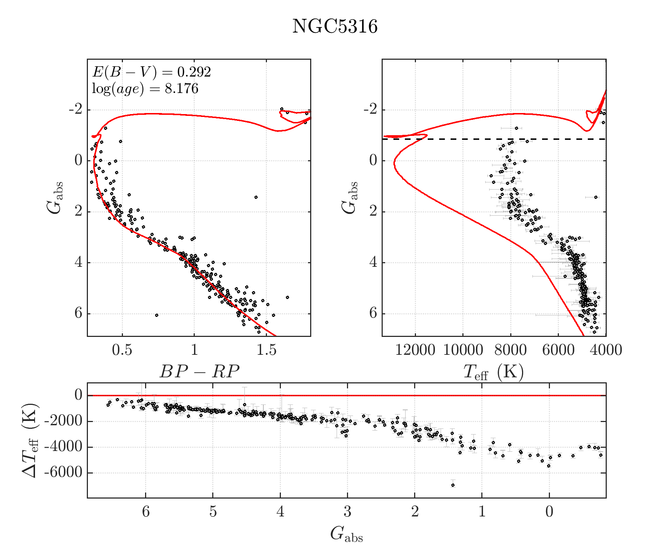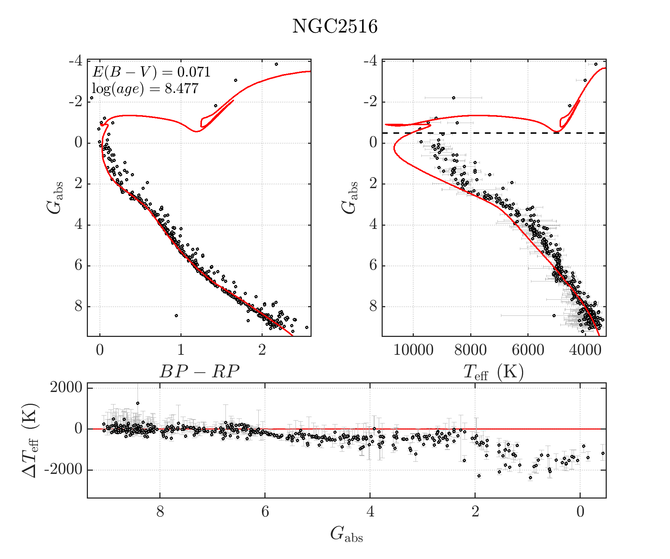# 10.7.5 Temperature

We compared Gaia the absolute magnitudes $G_{\mathrm{abs}}$ and effective temperatures $T_{\rm eff}$ with PARSEC isochrones (Chen et al. 2014) for about 180 clusters. In the regions of low extinction, we generally find a good agreement between the Gaia $T_{\rm eff}$ and the value expected using an isochrone of corresponding age (taken from Kharchenko et al. 2013), for stars $T_{\rm eff}\sim 7000-8000K$. The distribution of temperatures for NGC 2360 ($E(B-V)=0.07$) is shown in Figure 10.55. The underestimated temperatures are consistent with the fact that $T_{\rm eff}$ was derived under the assumption of $A_{\rm G}$=0. Stronger deviations are observed in the presence of moderate and high extinction, for instance for NGC 5316 (Figure 10.56), a cluster for which $E(B-V)=0.29$.

The $T_{\rm eff}$ values of Gaia DR2 were derived with a training set of templates in the range 3030 K $T_{\rm eff}<$ 9990 K (Andrae et al. 2018), restricting the output values to this range. This saturation effect is in fact seen even for temperatures lower than 9990 K, for instance NGC 2516 (Figure 10.57)where stars expected to be hotter than $T_{\rm eff}\sim 8000$ K already have underestimated temperatures. A certain granularity is also visible Figure 10.57, where points accumulate near certain values of $T_{\rm eff}$. This artefact is a consequence of the inhomogeneous distribution of templates in the training data.Figure 10.55: Gaia DR2 Teff (dots) compared with the expectations from a PARSEC isochrone (red line) for NGC 2360 on the Gabs versus GBP-GRP plane (left upper panel); on the Gabs versus Teff plane (right upper panel), and Δ⁢Teff versus Ga⁢b⁢s (lower panel) (from Arenou et al. 2018).Figure 10.56: The analogous of Figure 10.55. Gaia DR2 Teff for NGC 5316 (from Arenou et al. 2018).Figure 10.57: The analogous of Figure 10.55. Gaia DR2 Teff for NGC 2516 (from Arenou et al. 2018).